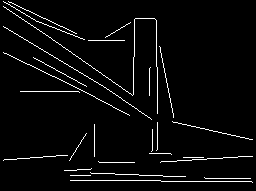# Straight Line

The Straight Line module provides a way to filter out straight lines from an image. This is functionally similar to the Hough Transform but will operate at a faster speed.

The Straight Line module expects an edge extracted image to work correctly. Thus you must use Canny or other edge finding modules before running the Line Corner module.

## Interface## Instructions

1. Be sure to have an edge detected image (using something like Canny) before using the Line Corner detection module.

2. Allowed Line Error - as the module follows the edge contours the allowed line error will ensure that the creation of a straight line does not deviate from the allowed error. Decreasing this number will create straight lines that better match the contour, larger values will allow the straight line to cover more of the line at the expense of accuracy.

3.Min Max Length - removes lines that are shorter than min length and longer than max length.

4. Angle Filter - removes lines that are not within min and max angle. These are values in degrees. For example, setting min to 85 and max to 95 would remove all lines that are not vertical.

5. Merge Like Lines - concatenates lines that are similar in slope and whose endpoints are relatively close.

6. Max Distance - the maximum distance of line endpoints that will be merged. The smaller this number the closer the line endpoints need to be in order to be merged into a single line. The higher this number the more distant the lines can be.

7. Merge Error - when comparing the slope of the two lines the merge error dictates how unlike the two slopes can differ to still be merged. Lowering this number will cause lines to have very similar slopes in order to be merged. Increasing this number will cause lines that are less like in slope to be merged. Note that the final merged line will have a combination of both line's slopes.

8. Extend Lines to Closest Intersection - As lines can be broken short of their expected end the "Extend Lines" will extend the line to the closest intersection to help complete the lines path.

9. Intersect Distance - the maximum distance any line intersection that will be considered. Increasing this number will extend lines further to local intersections. Decreasing this number will require intersections be closer to the actual end of both lines in order for an intersection to cause line extending.

10. Intersect Angle - when two lines intersect and angle between them is formed. This number will ensure that extended lines are only extended if the nearest intersection forms an angle that is above the number specified here. Increasing this number will cause lines to have a more perpendicular intersection in order to be extended. Decreasing this number will allow more and more parallel lines to be extended to their nearest intersection.

11. Parallel Lines Only - used to eliminate any line that does not have a parallel line nearby. This is handy when a group of parallel lines is to be extracted from an image. Note that parallel lines will be identified in pairs.

12. Overlap - specifies how similar in length the two overlapping parallel lines need to be in order to be considered a parallel line. Increasing this number will require more of the two lines to overlap in space, decreasing the number will allow parallel lines that slightly overlap each other to be considered.

13. Max Distance - the maximum distance the two parallel lines need to be before being considered parallel. Increasing this number will allow parallel lines that are spatial further away from each other to be considered parallel. Decreasing this number will force them to be closer together.

14. Create STRAIGHT_LINE Array - creates a VBScript accessible array that holds the start and end coordinates of any resulting line. The first line start coordinate is STRAIGHT_LINE(0) as the X axis, STRAIGHT_LINE(1) as the Y axis, and STRAIGHT_LINE(2), STRAIGHT_LINE(3) being the endpoint of the line. The second line start point would then be STRAIGHT_LINE(4), STRAIGHT_LINE(5), and so on.

## Example

 Source Canny Edge DetectionStraight Lines Detected (>5 length) Straight Lines Detected (>30 length)Notice that some of the "straight" lines are in fact not perfectly straight in the original image. This is permitted due to the allowed error being high which can be made very lenient in what it considers to be a straight line.

## Variables

```STRAIGHT_LINE - the xstart, ystart, xend, yend points of all detected lines
in the current image.
```